# Math in Focus Grade 7 Chapter 4 Lesson 4.3 Answer Key Real-World Problems: Algebraic Equations

Practice the problems of Math in Focus Grade 7 Workbook Answer Key Chapter 4 Lesson 4.3 Real-World Problems: Algebraic Equations to score better marks in the exam.

## Math in Focus Grade 7 Course 2 A Chapter 4 Lesson 4.3 Answer Key Real-World Problems: Algebraic Equations

### Math in Focus Grade 7 Chapter 4 Lesson 4.3 Guided Practice Answer Key

Solve. Copy and complete.

Question 1.
Mark wrote a riddle: A negative number is $$\frac{2}{5}$$ of another negative number. If the sum of the two negative numbers is -35, find the two negative numbers.
Method 1
Use bar models.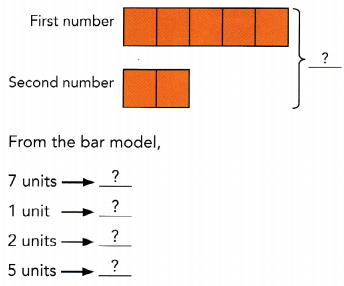The two negative numbers are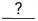and.

Method 2
Use algebraic reasoning.
Let one of the numbers be x.
Then the other number is $$\frac{2}{5}$$x.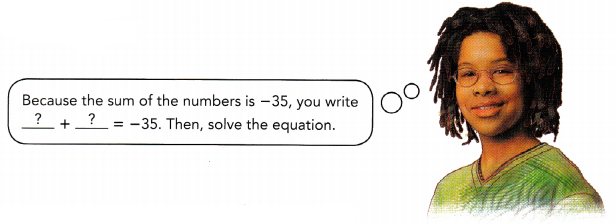+= -35 Write an equation.x = -35 Add the like terms.x ÷=÷Divide both sides byx =Simplify.
The other number: $$\frac{2}{5}$$x = $$\frac{2}{5}$$ ∙Evaluate $$\frac{2}{5}$$x when x =.
=The two negative numbers areand.
x = -25, (2/5)x = -10

Explanation:
Let one of the numbers be x.
Then the other number is (2/5)x.

x + (2/5)x = -35

(5x + 2x)/5 = -35

7x/5 = -35

Divide both sides by 7

7x/5 ÷ 7 = -(35) ÷ 7

(x/5) = -5

Multiply both sides by 5

(x/5) × 5 = -5 × 5

x = -25

(2/5)x = (2/5) × (-25)

= 2 × -5

= -10

Solve.

Question 2.
At an auditorium, tickets are sold for “circle seats” and “row seats.” There are 220 circle seats, and the rest of the seats are row seats. Each circle seat ticket costs $100 and each row seat ticket costs$60.
a) Write an expression for the total amount collected from the sale of all the seats at the auditorium.
100(220) + 60(y – 220) = x

Explanation:
Let us consider the amount collected from the sale be ‘x’

Number of seats be ‘y’

Number of circle seats =220

Number of row seats = y – 220

Cost of each circle seat = $100 Cost of each row seat =$60

100(220) + 60(y – 220) = x

b) The total amount collected when all the tickets are sold is $68,800. How many row seat tickets are sold? Answer: x = 780 Explanation: Number of row tickets be ‘x’ The total amount collected when all the tickets are sold =$68,800

With the above given details

100(220) + 60(x) = 68800

22000 + 60x =68800

subtract 22000 on both sides.

22000 + 60x – 22000 =68800 – 22000

60x = 46800

Divide 6 on both sides.

60x ÷ 60 = 46800 ÷ 60

x = 780

Copy and complete eachwith a value and each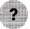with +, -, ×, or ÷.

Question 3.
James has 16 more game cards than Fay. If they have 48 game cards altogether, find the number of game cards James has.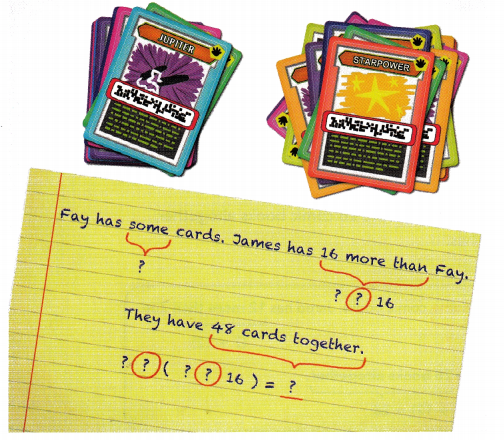Let the number of cards that Fay has be x.
Then the number of cards that James has is x16.
Because they have 48 cards altogether,
x(x16) =x16 –x16=x =x=x =Number of cards that James has:
x1616
=James hasgame cards.
James has 32 game cards

Explanation:
Let the number of cards that Fay has be x.

Then the number of cards that James has is x + 16

Because they have 48 cards altogether,

x + x+ 16 = 48

2x + 16 = 48

Subtract 16 on both sides.

2x + 16 – 16 = 48 -16

2x = 32

Divide 2 on both sides

2x ÷ 2 = 32 ÷ 2

x = 16

number of cards that Fay, x = 16

the number of game cards James has x + 16 = 16 + 16

### Math in Focus Course 2A Practice 4.3 Answer Key

Question 1.
Two sections of a garden are shaped like identical isosceles triangles. The base of each triangle is 50 feet, and the other two sides are each x feet long. If the combined perimeter of both gardens is 242 feet, find the value of x.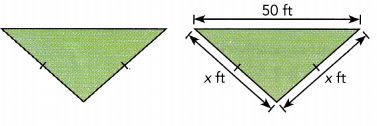a = 35.5

Explanation:
Let us consider the sides be x

The perimeter of both the gardens = 242

2 × garden areas = 242

one garden are perimeter = 242 ÷ 2

one garden are perimeter = 121

Perimeter of a isosceles triangle = 2a +b

b =50

2a + 50 =121

Subtract 50 on both sides.

2a +50 – 50= 121 -50

2a = 71

Divide 2 on both sides

2a ÷ 2 = 71 ÷ 2

a = 35.5

Question 2.
Richard has a rectangular plot of land that is 525 feet long and y feet wide. He decides to build a fence around the plot. If the perimeter of the plot is 1,504 feet, find the value of y.
y = 227

Explanation:
Given, perimeter of the plot = 1504

Length of the plot, l = 525

Breadth of the plot, b = y

Perimeter of the plot = 2(l + b)

1504 = 2 (525 + y)

Divide 2 on the both sides

1504 ÷ 2 = 2 (525 + y)÷ 2

752 = 525 + y

Subtract 525 on both sides.

752 – 525 = 525 + y -525

y = 227

Question 3.
The diagram shows an artificial lake. When Amanda jogged twice around the lake, she jogged a distance of 2,700 meters. Find the value of x.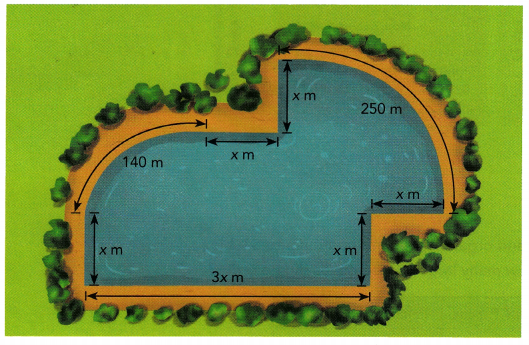x = 120

Explanation:

As Amanda jogged twice around the lake.

2(x + 140 + x + x+ 250+x +x + 3x) = 2700

2 (8x + 390) = 2700

Divide 2 on both sides

2 (8x + 390) ÷ 2 = 2700 ÷ 2

8x + 390 = 1350

Subtract 390 on both sides.

8x + 390 – 390 = 1350 -390

8x = 960

Divide 8 on both sides

8x ÷ 8= 960 ÷ 8

x = 120

Question 4.
Olivia wants to trim a lampshade with braid. The lampshade is shaped like a rectangular prism. The length of the base of the lampshade is 4 inches greater than its width. If the perimeter of the base is 54 inches, find the length of the base.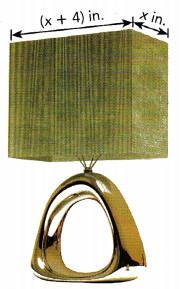Explanation:
Given, perimeter of the base = 54 inches

Consider length of the lamp, l = (x +4)

x = (l – 4)

Consider breadth of the lamp, b = l

perimeter of the base = 54

2 (l + b) = 54

2 ((l – 4) + l) = 54

Divide 2 on both sides.

2 ((l – 4) + l) ÷ 2 = 54 ÷ 2

2l – 4 =  27

2l – 4 + 4 =  27 + 4

2l = 31

Divide 2 on both sides

2l ÷ 2 = 31 ÷ 2

l = 15.5

Length, x = l -4 = 15.5 – 4 = 11.5

Verification:
2(l + b ) = 54

2(11.5 + 15.5)

2 (27)

54

Hence proved

Question 5.
Daphne was given a riddle to solve: The sum of two consecutive positive integers is 71. Find the two positive integers.

Explanation:
Consider the two consecutive positive numbers be x, x +1

Given, The sum of two consecutive positive integers = 71

x + x +1 = 71

2x +1 = 71

Subtract 1 on both sides.

2x +1 -1 = 71 – 1

2x = 70

Divide 2 on both sides.

2x ÷ 2 = 70 ÷ 2

x = 35

since, x =35  ==>  x + 1 = 35 + 1 = 36

Verification:
x + x +1 = 71

35 + 36 = 71

Hence proved.

Question 6.
The sum of a negative number, $$\frac{1}{4}$$ of the negative number, and $$\frac{7}{16}$$ of the negative number is -13 $$\frac{1}{2}$$. What is the negative number?
-x = -9 (5/11)

Explanation:
-x(1/4) – x(7/16) =  -13 (1/2)

-11x/16 = -13(1/2)

Multiply 2 on both sides.

(-11x/16) × 2 = -13(1/2) × 2

(-11x/8) = -13

Multiply 8 on both sides.

(-11x/8) × 8 =-13 × 8

x = 9 (5/11)

Question 7.
3.5 times a positive number is equal to the sum of the positive number and 0.5. What is the positive number?
x = 0.2

Explanation:
Let us consider the positive number be ‘x’

3.5x = x + 0.5

Subtract x on both sides.

3.5x – x = x + 0.5 – x

2.5x = 0.5

Divide 2.5 on both sides.

2.5x ÷ 2.5 = 0.5 ÷ 2.5

x = 0.2

Verification:

Positive Number x = 0.2

3.5x = x + 0.5

Substitute 0.2 in the equation.

3.5(0.2) = 0.2 + 0.5

0.7 = 0.7

Hence Proved.

Question 8.
Eugene wrote a riddle: A positive number is 5 less than another positive number. Six times the lesser number minus 3 times the greater number is 3. Find the two positive numbers.
x = 11

Explanation:
Let us consider the two positive numbers be  ‘x’ and  ‘x -5’

Given, Six times the lesser number minus 3 times the greater number is 3

6 (x – 5) – 3(x) = 3

6x – 30 -3x = 3

3x – 30 = 3

3x – 30 + 30 = 3 + 30

3x = 33

Divide 3 on both sides.

3x ÷ 3 = 33 ÷ 3

x = 11

Verification:
6 (x – 5) – 3(x) = 3

Substitute x = 11

6 (11 – 5) – 3(11)

= 6 × 6 – 33

= 36 – 33

= 3

Hence proved.

Question 9.
At a charity basketball game, 450 tickets were sold to students at a school. The remaining x tickets were sold to the public. The prices of the two types of tickets are shown. When all the tickets were sold, $10,500 was collected. How many tickets were sold to the public?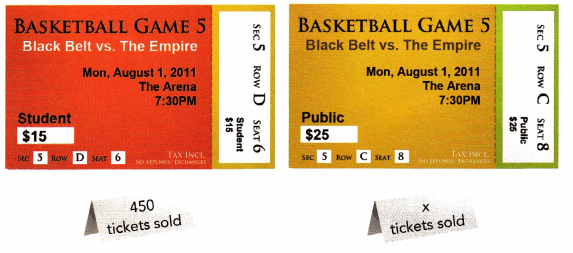Answer: x = 150 Explanation: Consider the number of tickets sold to public be ‘x’ The cost of each ticket sold to the student = 15$

Number of students at school the tickets were sold = 450

Cost of ticket to the public = 25$Total amount collected when all the tickets were sold =$10,500

25(x) + 15(450) = 10500

25x + 6750 = 10500

Subtract 6750 on both sides.

25x + 6750 – 6750 = 10500 – 6750

25x = 3750

Divide 25 on both sides.

25x ÷ 25 = 3750 ÷ 25

x = 150

Verification:
25(x) + 15(450) = 10500

Substitute x = 150

25(x) + 15(450)

= 25(150) + 15(450)

= 3750 + 6750

= 10,500

Hence proved.

Question 10.
Henry ordered pizzas for a party and organized the information into a table. If Henry paid a total of $93.65, how many large cheese pizzas did he order?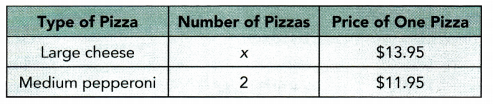Answer: Explanation: Total amount Henry paid = 93.65$

Number of medium pepperoni pizza = 2

Price of one medium pepperoni pizza = $11.95 Number of large cheese be ‘x’ Price of one large cheese =$13.95

2(11.95) + x(13.95) = 93.65

23.9 + 13.95x = 93.65

Subtract 23.9 on both sides.

23.9 + 13.95x – 23.9 = 93.65 – 23.9

13.95x = 69.75

Divide 13.95 on both sides.

13.95x ÷ 13.95 = 69.75 ÷ 13.95

x = 5

Verification:
2(11.95) + x(13.95) = 93.65

23.9 + 13.95x

Substitute x = 5

23.9 + 13.95(5)

= 23.9 + 69.75

= 93.65

Hence proved.

Question 11.
Marvin saved dimes and quarters in his piggy bank to buy a gift for his mother. He counted his savings and organized the information in a table.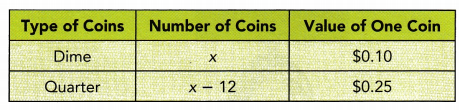If he saved $11, how many dimes and quarters did Marvin have? Answer: Dime, x = 40 ; Quarter, x- 12 = 28 Explanation: Given, Number of dime coins = x Number of quarter coins = x – 12 Value of one dime coin = 0.10$

Value of one quarter coin = 0.25$0.10x + 0.25(x – 12) =11 0.10x + 0.25x – 3 =11 Add 3 on both sides. 0.10x + 0.25x – 3 + 3 =11 + 3 0.35x =14 Divide 0.35 on both sides. 0.35x ÷ 0.35 =14 ÷ 0.35 x = 40 x – 12 = 40 -12 = 28 Verification: 0.10x + 0.25(x – 12) =11 Substitute x = 40 0.10(40)+ 0.25(40- 12) = 4 + 7 = 11 Question 12. A bike shop charges x dollars to rent a bike for half a day. It charges (x + 40) dollars to rent a bike for a full day. The table shows the shop’s bike rentals for one day. On that day, the shop made a total of$600 from bike rentals.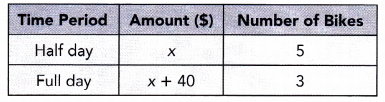How much does it cost to rent a bike for a full day?
Amount for full day = x + 40 =  60 + 40 = 100$Explanation: Given, Amount for half day = x Number of bikes taken for rental for half day = 5 Amount for full day = x + 40 Number of bikes taken for rental for full day = 3 Total number of amount the bike shop made on rental on that day =$600

5(x) + 3(x +40) = 600

5x + 3x +120 = 600

Subtract 120 on both sides.

5x + 3x +120 – 120 = 600 – 120

8x = 480

Divide 8 on both sides.

8x ÷ 8 = 480 ÷ 8

x = 60

Amount for full day = x + 40 = 60 + 40 = 100

Verification:
5(x) + 3(x +40) = 600

= 5x + 3x +120

= 8x + 120

Substitute x = 60

8 (60) +120

= 480 + 120

= 600

Hence proved

Question 13.
An artist is weaving a rectangular wall hanging. The wall hanging is already 18 inches long, and the artist plans to weave an additional 2 inches each day. The finished wall hanging will be 60 inches long. How many days will it take the artist to finish the wall hanging?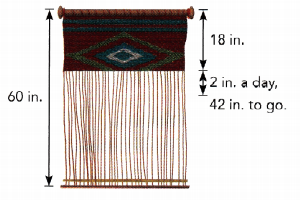x = 21 days

Explanation:
Given, total length of the wall hanging = 60 inches

Artist finished 18 inches, so the remaining would be 60 – 18 = 42

the artist plans to weave an additional 2 inches each day

i.e; 2 inches – 1 day

42 inches – ?

Let us consider the no. of days for weaving 42 inches be ‘x’

2 inches – 1 day

42 inches – x days

Let us cross multiply

2 × (x) = 42 × 1

2x = 42

Divide 2 on both sides.

2x ÷ 2 = 42 ÷ 2

x = 21 days

Question 14.
Ms. Kendrick plans to buy a laptop for $1,345 in 12 weeks.$he has already saved $145. How much should she save each week so she can buy the laptop? Answer: Amount she should save each week, x = 100 Explanation: Given, Ms. Kendrick plans to buy a laptop for$1,345 in 12 weeks

she already saved $145. Let us consider the amount per week in the 12 weeks = x 12x + 145 = 1345 Subtract 145 in both sides. 12x + 145 – 145 = 1345 – 145 12x = 1200 Divide 12 on both sides. 12x ÷ 12 = 1200 ÷ 12 x = 100 Question 15. A plant grows at a rate of 4.5 centimeters per week. It is now 12 centimeters tall. 5uppose that the plant continues to grow at the same rate. In how many weeks will it reach a height of 48 centimeters? Answer: x = 8 weeks Explanation: We can apply the formula, y = mx + b y = 48 m = 4.5 b= 12 48 = 4.5x + 12 Subtract 12 on both sides. 48 – 12 = 4.5x + 12 – 12 4.5x = 36 Divide 4.5 on both sides. 4.5x ÷ 4.5 = 36 ÷ 4.5 x = 8 Question 16. Mr. Johnson is currently four times as old as his son, David. If Mr. Johnson was 46 years old two years ago, how old is David now? Answer: David age, x = 12 Explanation: Let us consider, David’s age be ‘x’ Mr. Johnson is currently four times as old as his son, s0 4x As Mr. Johnson was 46 years old two years ago, at present his age is 46 +2 = 48 4x = 48 Divide 4 on both sides 4x ÷ 4 = 48 ÷ 4 x = 12 Question 17. Mr. Warren drove from Townsville to Villaville and back again at the speeds shown. His total driving time was 12 hours. How far apart are the two towns?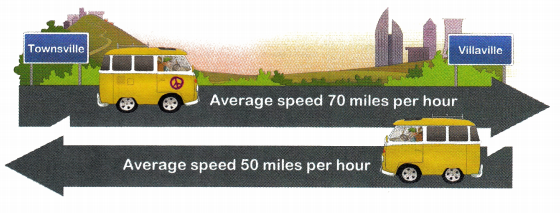Answer: Distance, x = 350 miles Explanation: Distance from townsville to villaville = x/70 miles per hour Distance from villavile to townsville = x/50 miles per hour it takes 12 hours x/70 + x/50 = 12 (7x + 5x)/350 = 12 12x/350 = 12 Divide 12 on both sides. 12x/350 ÷ 12 = 12 ÷ 12 x/350 = 1 x = 350 miles Question 18. A factory made 845 pairs of shoes in January. These shoes were sent to three shoe stores and one outlet mall. The number of pairs of shoes sent to each store was four times the number sent to the outlet mall. How many pairs of shoes were sent to the outlet mall in January? Answer: Pairs of shoes were sent to the outlet mall in January, x = 65 Explanation: Consider the number of pairs shoes sent to outlet mall be ‘x’ Number of pairs shoes sent to each store = 4x + 4x +4x 4x + 4x +4x +x = 845 13x =845 Divide 13 on both sides 13x ÷ 13 =845 ÷ 13 x = 65 Question 19. The cost of seeing a weekday show is $$\frac{2}{3}$$ the cost of a weekend show. In one month, Andy spent$42.50 for 4 weekday shows and 3 weekend shows. Find the price of a weekday show and the price of a weekend show.
The cost of seeing a weekday show, x = 5

The cost of seeing weekend show, y = 7.5

Explanation:
Let us consider, x be the cost of week day show

y be the cost of weekend show

x = (2/3)y

In one month, Andy spent \$42.50 for 4 weekday shows and 3 weekend shows

4x + 3y = 42.50

4(2/3)y + 3y = 42.50

Multiply 3 on both sides

4(2/3)y × 3+ 3y × 3 = 42.50 × 3

8y + 9y = 127.5

17y = 127.5

Divide 17 on both sides

17y ÷ 17 = 127.5 ÷ 17

y = 7.5

x = (2/3)y = (2/3) × 7.5

= 5